# Support of a generalized function

(diff) ← Older revision | Latest revision (diff) | Newer revision → (diff)

The set of those (and only those) points such that in any neighbourhood of them the generalized function does not vanish. A generalized functioninvanishes in an open set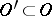iffor all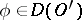. Using a partition of unity it can be proved that if a generalized functioninvanishes in some neighbourhoodfor each point, thenvanishes in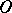. The union of all neighbourhoods in whichvanishes is called the zero set ofand is denoted by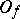. The support of, denoted by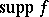, is the complement ofin, that is,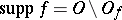is a closed set in. Ifis a continuous function in, then an equivalent definition of the support ofis the following:is the closure inof the complement of the set of points at whichvanishes (cf. Support of a function). For example,,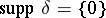.

The singular support (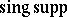) of a generalized function is the set of those (and only those) points such that in any neighbourhood of them the generalized function is not equal to a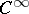-function. For example,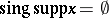,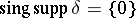.

The notion of a zero set as used above is somewhat unusual and does not agree with the zero set of an ordinary function (not a generalized function) as the set of points where that function assumes the value zero. Of course, the statement "fx=0" has no meaning for generalized functions.
A pointin the support of a generalized functionis called an essential point of, cf. [a4].